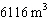Name:    Geometry Chapter 12 MC Practice

1.

Find the number of vertices, faces, and edges for the figure below.a. 3 vertices, 4 faces, 6 edges b. 5 vertices, 5 faces, 7 edges c. 4 vertices, 4 faces, 6 edges d. 6 vertices, 4 faces, 4 edges

2.

Find the surface area of the triangular prism.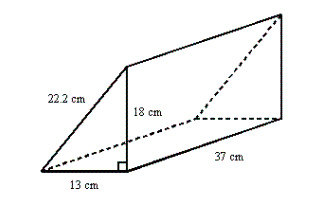a. 192,207.6 cm2 b. 2202.4 cm2 c. 1101.2 cm2 d. 3854.2 cm2

3.

Find the surface area of the cylinder to the nearest square unit. (Useand round the result to one decimal place.)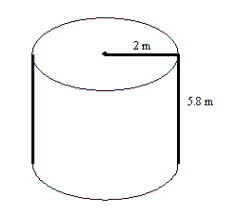a. 49 m2 b. 98 m2 c. 12 m2 d. 16 m2

4.

What is the surface area of a sphere with radius 4.7 feet?
 a. 434.9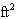b. 69.4c. 92.5d. 277.65.

Find the volume of a sphere 4 ft in diameter. Useand round your answer to the nearest tenth.
 a. 25.1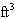b. 33.5c. 18.8d. 16.76.

Find the surface area of the right cone. (Use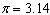and round the result to one decimal place.)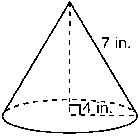a.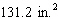b.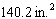c.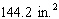d.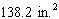7.

Find the volume of the right triangular prism.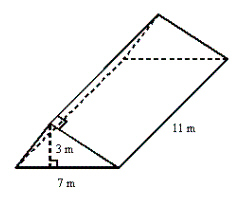a. 115.5b. 231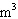c. 21.5d. 328.

Calculate the volume of the cone. (Useand round the result to TWO decimal places.)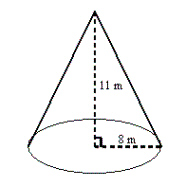a. 2210.56b. 736.85c. 234.67d. 66.999.

The pyramid shown has a square base and a slant height of 7 ft. Find its surface area.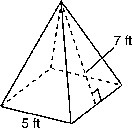a. 125c. 155b. 175d. 9510.

The pyramid shown has a rectangular base and faces that are isosceles triangles. Find its volume.a.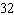c. 37b. 23d. 2711.

Find the volume of the cylinder. (Useand round the result to one decimal place.)a.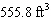c.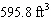b.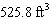d.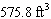12.

Find the volume of the rectangular prism.a.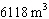c.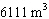b.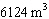d.